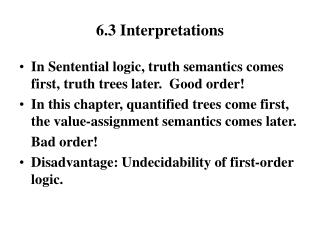DownloadDownload Presentation6.3 Interpretations

# 6.3 Interpretations

Download Presentation## 6.3 Interpretations

- - - - - - - - - - - - - - - - - - - - - - - - - - - E N D - - - - - - - - - - - - - - - - - - - - - - - - - - -
##### Presentation Transcript

1. 6.3 Interpretations • In Sentential logic, truth semantics comes first, truth trees later. Good order! • In this chapter, quantified trees come first, the value-assignment semantics comes later. Bad order! • Disadvantage: Undecidability of first-order logic.

2. Definitions • An interpretationmaking a set of formulas true is a model of the set. • An interpretation M of a set S of formulas of QL is an ordered set < D, [] >, where D is M’s nonempty domain , and [] is a function assigning: • truth values to sentential letters in S • elements of D to constants in S • sets of n-tuples of elements of D to n-ary predicates in S

3. Two Semantic Components • D: General individual domain for x (not quantifier) to range over. • []: Value-assignment function: • Sentential letter truth value • constant object in D • unitary predicate subset of D • binary predicate subset of pairs in DxD • n-ary predicate subset of n-tuples Dn

4. Truth Conditions by M • [p]m = T iff p] = T • [Ra1 …an]m=T iff <[a1], …, [an]> is in [R] • [¬A]m = T iff [A]m = F • [(A&B)]m = T iff [A]m=[B]m=T • [(AVB)]m = T iff [A]m=T or [B]m=T • [(A→B)]m = T iff [A]m=F or [B]m=T • [(A↔B)]m = T iff [A]m=[B]m

5. Truth Conditions for Quantifiers • c-variant M’ of M, just like M but [ ]’may assign different object to c • [uA]m = T iff [Ac/u]m’ = T for some c-variant M’ of M (there is some [a] in D s.t. A(a) = T) • [uA]m = T iff [Ac/u]m’ = T for every c-variant M’ of M (every [a] in D s.t. A(a) = T)

6. 6.4 Constructing Interpretations from Trees • Consider only a finished, open tree • Consider an open branch including nodes: Fab, Fbb, Ga, ¬Gb, ¬Faa The model M = < D, [ ] > is [F]={<a, b>, <b, b>}, [G]={<a>}, D = {a, b}

7. Rules • D = {a1, …, an, …}, where a1, an, … are the constants appearing in atomics on the open branch. • [p] = T if p is on the branch; F, otherwise. • [R] = {<a1, …, an>, <b1, …, bn>, …}, where R(a1, …an), R(b1, …,bn), and so on, appear on the branch.